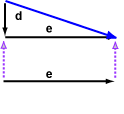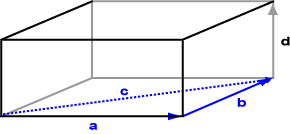The diagram shows the vector e sliding up until its tail touches the head of d.

The sum is the vector from the tail of d to the head of e .

# Vector Addition in 3D

The diagram shows 3D vectors a and b added to form c (the 3D box is there to aid in visualizing the three dimensions). The head-to-tail rule works in 3D as well.When two vectors are added in 3D, the head-to-tail rule defines a triangle oriented in 3D space.

### QUESTION 5:

Is it possible (do you think) to add three vectors together, like a+b+d in the figure?